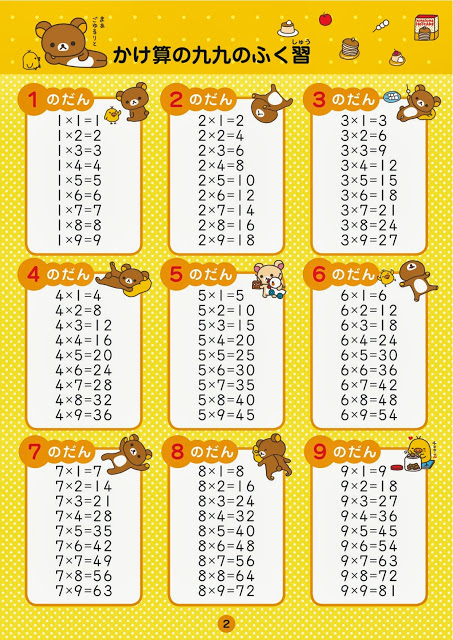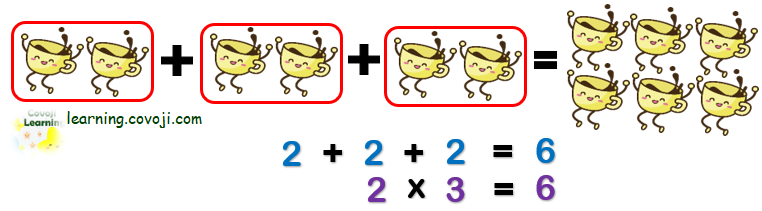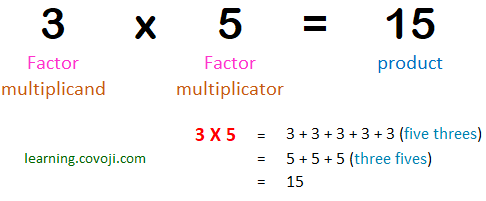The basic idea of multiplication is repeated addition. To multiply means to add equal groups.Sign For Multiplication

The most common is the “x” sign, but sometimes people use a “*”.

• 3 x 6
• 3 * 6
• 3 times 6
• 3 multiplied by 6

#### To Remember

• Any number multiplied by zero is also zero: 4 x 0 = 0.
• Any number multiplied by one results in the same value. 8 x 1 = 8.
• Order does not matter. You can multiply the number in any order you want and the answer will be the same. 6 x 3 = 3 x 6 = 18.
• Double the other factor when multiplied by two. 6 x 2 = 12. Just double 6 to make 12!Categories: Math Zone